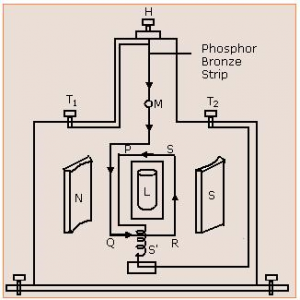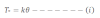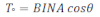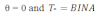# Moving Coil Galvanometer

It is a device that is used to measure and detect the small amount of electric charge i.e. electric current. It is the most sensitive device and used in many electrical instruments. It is the major basic component of Ammeter and Voltmeter.

Principle:
Moving coil galvanometer is based on the fact that when a current-carrying rectangular coil is placed in a magnetic field it experiences torque.Construction:
Construction of the galvanometer is as shown in the figure. In the galvanometer, it consists of rectangular coil PQRS having N number of an insulated copper coil which is wounded in the nonmagnetic metallic frame of soft iron as a center core. The coil is suspended by a phosphor-bronze strip through a torsion head to that it is free to rotate about the vertical axis. The coil is placed in between two pieces of magnet. The other end of the coil is connected to the light spring and finally, to the terminal T2 The torsion head is connected to the terminal T1. A concave mirror m is placed to the suspension strip to note the deflection of the coil using a lamp and scale arrangement.

Theory:
When a current I flows through the rectangular coil, the coil gets deflected, the suspension wire is twisted and rotating torque is developed on it. If k is rotating torque per unit angle per unit twist of the strip then restoring torque through the angle θ is:if A be the area of the rectangular strip, N be the number of turns, I be the current through the coil and B be the magnetic field then the torque on the rectangular coil is:Since the rectangular coil is placed in between two concave-shaped magnets then the field lines are radial to the area of the rectangular field. so the area of the rectangular foil is parallel to the magnetic field lines then,So the deflection of the galvanometer is directly proportional to the current on the rectangular coil. Hence we use a linear scale in the galvanometer.